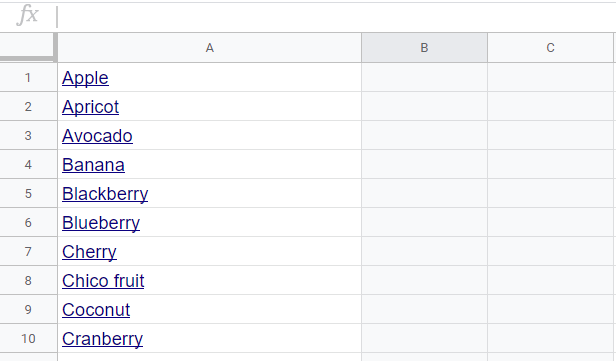# How to Randomly Pick a Name from A Long List in Google Sheets

A simple, step-by-step tutorial on how to randomly pick an entry from a list on Google Sheets. Uses the INDEX, RANDBETWEEN, and COUNTA functions in the process.Do you plan to run a raffle from a long list of names in your spreadsheet? Well, here is a quick solution you can implement in Google Sheets! Here are the steps:

Step 1: Open the workbook containing the long list of names to pick from. We will use this list of 41 fruits for this tutorial.The range of the cells containing the list is A1:A41. This is important for our next step.

Step 2: On the cell where you want to write down the selected entry, add this formula:

=index(<range_of_list>,RANDBETWEEN(1, <number_of_entries>))</number_of_entries></range_of_list>

Where <range_of_list> is the range of the cells containing the list and the <number_of_entries> is the number of entries contained in the list. </number_of_entries></range_of_list>

The RANDBETWEEN function randomly selects an integer from 1 to a specified maximum number. The maximum number should match the number of items in the list.

If you are not sure of how many entries are in the list but they are all in a single column, you can write the range as follows:

<first_cell_in_the_list>:<column_letter_of_the_list></column_letter_of_the_list></first_cell_in_the_list>

So for our example, we can write our range as A1:A, setting the first entry as A1 and then followed by all the cells with entries in column A. To replace <number_of_entries>, we can use the COUNTA function, defining its range in the same manner:</number_of_entries>

COUNTA(<first_cell_in_the_list>:<column_letter_of_the_list>)</column_letter_of_the_list></first_cell_in_the_list>

Plugging them together, we can have

=index(<range_of_list>,RANDBETWEEN(1, COUNTA(<range_of_list>)))</range_of_list></range_of_list>

This equation is more flexible because it allows you to add or remove entries in the list!

Once you entered the formula, Google Sheets will then select an item from the list